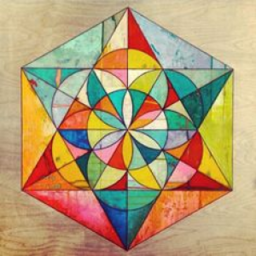# CCSS Gr. 8 Geometry

Topic:
Geometry
-------------------Under Construction--------------------- Common Core Standards for Grade 8-Geometry Understand congruence and similarity using physical models, transparencies, or geometry software. CCSS.Math.Content.8.G.A.1 Verify experimentally the properties of rotations, reflections, and translations: CCSS.Math.Content.8.G.A.1a Lines are taken to lines, and line segments to line segments of the same length. CCSS.Math.Content.8.G.A.1b Angles are taken to angles of the same measure. CCSS.Math.Content.8.G.A.1c Parallel lines are taken to parallel lines. CCSS.Math.Content.8.G.A.2 Understand that a two-dimensional figure is congruent to another if the second can be obtained from the first by a sequence of rotations, reflections, and translations; given two congruent figures, describe a sequence that exhibits the congruence between them. CCSS.Math.Content.8.G.A.3 Describe the effect of dilations, translations, rotations, and reflections on two-dimensional figures using coordinates. CCSS.Math.Content.8.G.A.4 Understand that a two-dimensional figure is similar to another if the second can be obtained from the first by a sequence of rotations, reflections, translations, and dilations; given two similar two-dimensional figures, describe a sequence that exhibits the similarity between them. CCSS.Math.Content.8.G.A.5 Use informal arguments to establish facts about the angle sum and exterior angle of triangles, about the angles created when parallel lines are cut by a transversal, and the angle-angle criterion for similarity of triangles. For example, arrange three copies of the same triangle so that the sum of the three angles appears to form a line, and give an argument in terms of transversals why this is so. Understand and apply the Pythagorean Theorem. CCSS.Math.Content.8.G.B.6 Explain a proof of the Pythagorean Theorem and its converse. CCSS.Math.Content.8.G.B.7 Apply the Pythagorean Theorem to determine unknown side lengths in right triangles in real-world and mathematical problems in two and three dimensions. CCSS.Math.Content.8.G.B.8 Apply the Pythagorean Theorem to find the distance between two points in a coordinate system. Solve real-world and mathematical problems involving volume of cylinders, cones, and spheres. CCSS.Math.Content.8.G.C.9 Know the formulas for the volumes of cones, cylinders, and spheres and use them to solve real-world and mathematical problems.